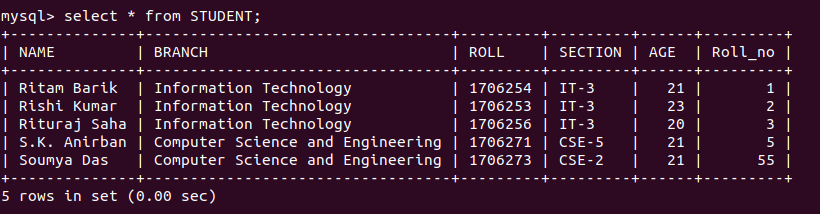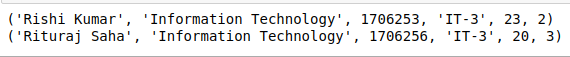# Python MySQL – Limit Clause

• Difficulty Level : Basic
• Last Updated : 18 Mar, 2020

A connector is employed when we have to use MySQL with other programming languages. The work of mysql-connector is to provide access to MySQL Driver to the the required language. Thus, it generates a connection between the programming language and MySQL Server.

#### Python-MySQL-Connector

This is a MySQL Connector that allows Python to access MySQL Driver and implement SQL queries in its programming facility. Here we will try implementing Limit clause on our Database and will study the output generated.

## LIMIT Clause Of SQL

The Limit clause is used in SQL to control or limit the number of records in the result set returned from the query generated. By default, SQL gives out the required number of records starting from the top but it allows the use of OFFSET keyword. OFFSET allows you to start from a custom row and get the required number of result rows.

Syntax:

```SELECT * FROM tablename LIMIT limit;

SELECT * FROM tablename LIMIT limit OFFSET offset;
```

DATABASE IN USE:Example 1: program to display only 2 records

 `import` `mysql.connector ` `  `  `# Conencting to the database ` `mydb ``=` `mysql.connector.connect( ` `  ``host ``=``'localhost'``, ` `  ``database ``=``'College'``, ` `  ``user ``=``'root'``, ` `) ` `  `  `cs ``=` `mydb.cursor() ` ` `  `# STUDENT and STudent are ` `# two different database ` `statement ``=``"SELECT * FROM STUDENT LIMIT 2"` ` `  `cs.execute(statement) ` `result_set ``=` `cs.fetchall() ` ` `  `for` `x ``in` `result_set: ` `    ``print``(x) `

OUTPUT:Example 2:program to start from the second record and display the first two records

 `import` `mysql.connector ` `  `  `# Conencting to the database ` `mydb ``=` `mysql.connector.connect( ` `  ``host ``=``'localhost'``, ` `  ``database ``=``'College'``, ` `  ``user ``=``'root'``, ` `) ` `  `  `cs ``=` `mydb.cursor() ` ` `  `# STUDENT and STudent are ` `# two different database ` `statement ``=``"SELECT * FROM STUDENT LIMIT 2 OFFSET 1"` ` `  `cs.execute(statement) ` `result_set ``=` `cs.fetchall() ` ` `  `for` `x ``in` `result_set: ` `    ``print``(x) `

OUTPUT:My Personal Notes arrow_drop_up
Recommended Articles
Page :